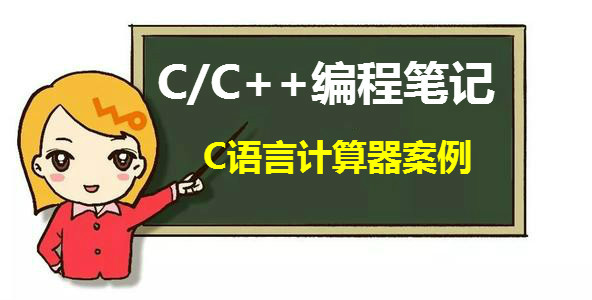• 完成一个简易计算器，具有加减乘除开方，平方，立方和退出功能的计算器。按照程序运行效果图，完成计算器。 ![图片说明](https://img-ask.csdn.net/upload/202005/16/1589596761_479682.png)c++
• 该楼层疑似违规已被系统折叠 隐藏此楼查看此楼 #include #... } } 还没测试过，做构思吧，我百度了一下，你去这地方看，那里跟你想要的更合适： http://wenku.baidu.com/view/b841d90003d8ce2f006623cf.html
该楼层疑似违规已被系统折叠 隐藏此楼查看此楼#include#include#define UINT unsigned intstruct LOGIC{UINT logic,site;};char *text="+8*9*(8+7)+12/6-4";int calc_exp(char *sz);int calc_part(int a,int b,UINT w);int main(int argc,char *argv[]){std::cout<std::cin.get();return 0;}int calc_exp(char *sz){int calc_rt=0;UINT szcnt=0;LOGIC *logic;UINT *expval;UINT lcnt=0;UINT ecnt=0;bool bj=false;UINT ti=0;int tn=0;int tr=0;char *tch=0;szcnt=sizeof(sz);bj=false;for(UINT i=0;i{switch(sz[i]){case '+':case '-':case '*':case '/':case '(':case ')':bj=false;lcnt++;break;default:if(bj==false){ecnt++;bj=true;}break;}}if(lcnt!=0)logic=new LOGIC[lcnt];if(ecnt!=0)expval=new UINT[ecnt];else return 0;bj=false;ti=0;tn=0;for(UINT i=0;i{switch(sz[i]){case '+':case '-':case '*':case '/':case '(':case ')':switch(sz[i]){case '+':logic[ti].logic=0;break;case '-':logic[ti].logic=1;break;case '*':logic[ti].logic=2;break;case '/':logic[ti].logic=3;break;case '(':logic[ti].logic=4;break;case ')':logic[ti].logic=5;break;}bj=false;ti++;break;default:if(bj==false){if(tch!=0){delete[] tch;tch=0;}tch=new char[logic[ti].site-i];for(UINT j=i;j{tch[j-i]=sz[j];}expval[tn]=atoi(tch);tn++;bj=true;}break;}}if(tch!=0){delete[] tch;tch=0;}if(lcnt==0)return expval;ti=0;tn=-1;while(ti{switch(logic[ti].logic){case 0:case 1:if(tn!=-1){calc_part(calc_rt,tr,logic[tn].logic);tn=-1;}else{tn=ti;tr=expval[ti];}break;break;case 2:tr*=expval[ti];break;case 3:tr/=expval[ti];break;case 4:for(UINT j=ti;j{if(logic[j].logic==5){tch=new char[logic[j].site-logic[ti].site-1];for(UINT k=0;k{tch[k]=sz[logic[ti].site+1+k];}calc_part(tr,calc_exp(tch),logic[ti-1].logic);ti=j;break;}}break;}ti++;}return calc_rt;}int calc_part(int a,int b,UINT w){switch(w){case 0:return (a+b);break;case 1:return (a-b);break;case 2:return (a*b);break;case 3:return (a/b);break;}}还没测试过，做个构思吧，我百度了一下，你去这个地方看，那里跟你想要的更合适：http://wenku.baidu.com/view/b841d90003d8ce2f006623cf.html
展开全文• 该楼层疑似违规已被系统折叠隐藏此楼查看此楼#include#includechar Menu(void);float sum(float a,float b);float sub(float a,float b);float mul(float a,float b);float div(float a,float b);...
该楼层疑似违规已被系统折叠 隐藏此楼查看此楼#include#includechar Menu(void);float sum(float a,float b);float sub(float a,float b);float mul(float a,float b);float div(float a,float b);void main(){float a,b,r;char c;while(1){Menu();scanf("%c",&c);switch(c){case '1':scanf("%f%f",&a,&b);r= sum(a,b);printf("%f\n",r);break;case '2':scanf("%f%f",&a,&b);r= sub(a,b);printf("%f\n",r);break;case '3':scanf("%f%f",&a,&b);r= mul(a,b);printf("%f\n",r);break;case '4':scanf("%f%f",&a,&b);r= div(a,b);printf("%f\n",r);break;case '0':printf("谢谢使用 \n");return;}}system("pause");return 0;}char Menu(void){while(1){printf("       *-------------------------------*\n");printf("       |           请选择运算类型          \n");printf("       |-------------------------------|\n");printf("       |            1---加法             |\n");printf("       |            2---减法             |  \n");printf("       |            3---乘法             |\n");printf("       |            4---除法             |\n");printf("       |            0---退出             |\n");}}float sum(float a,float b){float num;num=a+b;return num;}float sub(float a,float b){float num;num=a-b;return num;}float mul(float a,float b){float num;num=a*b;return num;}float div(float a,float b){float num;num=a/b;return num;}
展开全文• 一个计算器程序 除了普通的计算，还支持简单的C语言（脚本）语句程序控制，包括变量代数计算，循环控制等。你用了就知道，原来做一个脚本语言解释器并不很难。
• 给定一个包含正整数、加(+)、减(-)、乘(*)、除(/)的算数表达式(括号除外)，计算其结果。 表达式仅包含非负整数，+， - ，*，/ 四种运算符和空格 。 整数除法仅保留整数部分。 示例 1: 输入: "3+2*2" 输出: 7...


给定一个包含正整数、加(+)、减(-)、乘(*)、除(/)的算数表达式(括号除外)，计算其结果。

表达式仅包含非负整数，+， - ，*，/ 四种运算符和空格  。 整数除法仅保留整数部分。

示例 1:

输入: "3+2*2"

输出: 7

示例 2:

输入: " 3/2 "

输出: 1

示例 3:

输入: " 3+5 / 2 "

输出: 5

#define MAX_DIGITAL_LEN 100000

int calculate(char* s){
int len = strlen(s);
char str[len];
for (int i = 0;  i < len; i++) {
str[i] = s[i];
}
int arrayDigital[MAX_DIGITAL_LEN] = {0};
char arrayString[MAX_DIGITAL_LEN];
int symbolNum = 0;
//数组存符号
for (int i = 0; i < len; i++) {
if (s[i] == '-' || s[i] == '+' || s[i] == '*' || s[i] == '/') {
arrayString[symbolNum++] = s[i];
}
}
char *symbol = "+-*/ ";
char *number = strtok(str, symbol);
int countNumber = 0;
//数组存数字
while (number) {
arrayDigital[countNumber++] = atoi(number);
number = strtok(NULL, symbol);
}
//减号并入数字
for (int i = 0; i < symbolNum; i++) {
if (arrayString[i] == '-') {
arrayDigital[i + 1] = -arrayDigital[i + 1];
}
}
//先乘除
for (int j = 0; j < symbolNum; j++) {
if (arrayString[j] == '*') {
arrayDigital[j + 1] = arrayDigital[j] * arrayDigital[j + 1];
arrayDigital[j] = 0;
} else if (arrayString[j] == '/') {
arrayDigital[j + 1] = arrayDigital[j] / arrayDigital[j + 1];
arrayDigital[j] = 0;
}
}
//后加减
int calResult = 0;
for (int k = 0; k < countNumber; k++) {
calResult += arrayDigital[k];
}
return calResult;
}

int main()
{
char *s = "3+2*2";
int result = calculate(s);
printf("%d", result);
system("pause");
return 0;
}


展开全文算法 字符串
• 本文案例用C语言一个简单的计算器，进行加、减、乘、除操作。本程序涉及的所有数学知识都很简单，但输入过程会增加复杂性。都是C语言很基础的东西（输入输出），学了之后能写出一个完整的C语言程序。适合涉及C语言...
大一学了C语言老师肯定会要求你们做一个这个课后作业！所以，先收藏是关键~本文案例用C语言做一个简单的计算器，进行加、减、乘、除操作。本程序涉及的所有数学知识都很简单，但输入过程会增加复杂性。都是C语言很基础的东西（输入输出），学了之后能写出一个完整的C语言程序。适合涉及C语言的基本语法，与输入与输出。适合初学者学习。

代码如下：

/*===============================================*\

** 设计目的：简单计算器，计算形如10*(20.2-30.6)+5.0/2的表达式值

** 简要原理：中缀式转逆波兰式（后缀式）

** IDE：Dev-Cpp 4.9.9.2

** 注意事项：括号必须是英文状态的

** 时间： 2020-9-05

\*===============================================*/

#include <stdio.h>

#include <string.h>

#include <stdlib.h>

#define maxn 1000

char buf[maxn], str[maxn], signStack[maxn], ch;

int len, id, idSign, idAns, i, n;

double ans[maxn];

void checkSign(char sign){

if(sign == '(') signStack[idSign++] = sign;

else if(sign == '*' || sign == '/'){

while(idSign && (signStack[idSign-1] == '*' || signStack[idSign-1] == '/'))

str[id++] = signStack[--idSign];

signStack[idSign++] = sign;

}else if(sign == ')'){

while(signStack[idSign-1] != '(') str[id++] = signStack[--idSign];

--idSign;

}else{

while(idSign && signStack[idSign-1] != '(') str[id++] = signStack[--idSign];

signStack[idSign++] = sign;

}

str[id++] = ' ';

}

int check(double a, char sign){

if(a == 0 && sign == '/'){

printf("除数不能为0!，程序结束。\n");

system("pause");

exit(EXIT_FAILURE);

}

return 1;

}

double cal(double a, double b, char sign){

switch(sign){

case '+': return a + b;

case '-': return a - b;

case '*': return a * b;

case '/': return a / b;

}

}

int main(){

printf("\t\t\t\t简单计算器\n\n");

printf("\t\t  ======================================\n");

printf("\t\t  ||  支持+ - * / ( ) 等符号和负数输入  ||\n");

printf("\t\t  ======================================\n");

printf("\t\t\t\t\t\t\n");

printf("\t\t\t\t\t\t完成时间：2020年9月5日\n");

printf("\t\t\t\t\t\t学习Q群：757874045\n");

printf("请输入一个表达式，如 -10*(20.2-30.6)+5.0/2\n以回车结束:\n");

do{

gets(buf); id = idSign = idAns = 0;

if((len = strlen(buf)) == 0) continue;

int flag = 0; //这个是用来判断'-'字符是负号还是减号的。1表示数字，0表示 +*/(

/*判断原理：如果第一次读取就碰到-，那么一定是负号，若最近一次读取是+*-/(那么

也一定是负号，如果是右括号或数字那么是减号*/

for(i = 0; i < len; ++i){

if(buf[i] == ' ') continue;

if(buf[i] >= '0' && buf[i] <= '9' || buf[i] == '.' || buf[i] == '-' && !flag){

str[id++] = buf[i]; flag = 1;

}

else {

str[id++] = ' '; checkSign(buf[i]);

if(buf[i] != ')') flag = 0;

else flag = 1;

}

}

while(idSign) str[id++] = signStack[--idSign];

for(i = 0, n = 0; i < id; ++i){

if(str[i] == ' ') continue;

/*用增加空格的方式判断‘-’字符是负号还是减号，若‘-’后面挨着数字或小数点，那么一定是负号*/

if(str[i] >= '0' && str[i] <= '9' || str[i] == '.' || str[i] == '-'

&& (str[i+1] >= '0' && str[i+1] <= '9' || str[i+1] == '.')){

sscanf(str + i, "%lf%n", &ans[idAns++], &n);

i += n - 1;  continue;

}else if(check(ans[idAns-1], str[i])){

ans[idAns-2] = cal(ans[idAns-2], ans[idAns-1], str[i]);

--idAns;

}

}

printf("结果是 %.2lf\n", ans);

printf("输入Y 继续, N 退出: ");

scanf("%s", ch);

}while(ch == 'Y' || ch == 'y');

printf("感谢您的使用！再见\n");

system("pause");

return 0;

}

迟早你会使用到的一个C语言案例，如果你是刚刚开学的小学弟，还没有开始学习C语言，那么你可以收藏一下！如果你是已经开始学了C语言的小伙伴，那么就希望本案例能够对你有帮助~

自学C/C++编程难度很大，如果你想更快提升自己的编程能力和编写项目的水平，欢迎一起共同成长！

C语言C++编程学习交流圈子，【点击进入】微信公众号：C语言编程学习基地

分享（源码、项目实战视频、项目笔记，基础入门教程）

欢迎转行和学习编程的伙伴，利用更多的资料学习成长比自己琢磨更快哦！

展开全文• //计算结果放在第一个操作数 op1 = getNextNum(); while(1) { opt = getOpt(); if ( opt == '=' ) break; op2 = getNextNum(); op1 = caculate(op1,op2,opt); } printf("%d\n",op1); } return 0; } 16分享举报
• 写在前面：这本书相当于给没有什么编译原理基础又想要做一个玩具的程序猿做的一个很浅的科普。但它确确实实能走一遍流程。 昨天翻了翻《龙书》，实在受不了，对菜逼太不友好了。网上一搜，有人推荐这本书。好在手头...
• 是否选择复利计算的输入格式： " %c" (注意：%c的前面有一个空格。输入的字符大小写皆可，即Y或y，N或n皆可) 输出格式："rate = %.4f, deposit = %.4f\n" 为避免出现格式错误，请直接拷贝粘贴题目中给的格式字符串和...
• 该楼层疑似违规已被系统折叠隐藏此楼查看此楼case 0x7b: dengyu=1; //key=11; //wei++; break; } while(temp!=0xf0) { temp=P3; temp=temp&0xf0; ...
• 之前用switch语句完成了一个简单计算器的功能，下面我给大家用if语句和while循环语句再实现一个简单计算器编程。要求： 实现简单的计算器，具体代码如下：#include <stdio.h> // 头文件void main() { char a = 0...while
• 题目计算器设计 学生姓名史昕雨 学号11102212 所在院计算机 专业网络工程 } 五课设总结 在做此次课设的过程中刚开始一点思绪都没有老师给的那么多题也不知道该怎么选择乍看貌似什么都不会做的感觉经过和同学商量...
• 题目如下：通过所学的知识（选择语句、循环语句）完成一个简单的计算器编程，要求不许抄袭，只能用自己所学的知识点，不可引用网上自己的不懂的程序。 要求的格式如下图： 要求以这样的一种格式进行输出，并能...switch
• 2.5 C语言编程流程   C语言的编程流程 a)解决什么问题 b)怎么解决 c)编写程序 d)分析结果         2. 解决什么问题 ？ 比如说：我们如何打开、关闭一个计算器呢？   3 那么我们怎么解决呢？    我们可以...
• 本文错发在另一个账号上，现在把他转过来。/*编写人@wonder版本：v0.1因为对这个游戏感兴趣，想到用编程求解法，固编写了本程序。本程序用来求游戏《计算器-游戏》的解法。输入：开始数字start，最大步数step，目标...
• 基础编程题目集7-12 两个数的简单计算器 (10 分)本题要求编写一个简单计算器程序，可根据输入的运算符，对2个整数进行加、减、乘、除或求余运算。题目保证输入和输出均不超过整型范围。输入格式：输入在一行中依次...
• 之前用switch语句完成了一个简单计算器的功能，下面我给大家用if语句和while循环语句再实现一个简单计算器编程。 要求： 实现简单的计算器，具体代码如下： #include <stdio> // 头文件 void main() { char a = ...
• 编程思路 我们可以输入段逆波兰表达式，然后程序循环读取单个字符，判断字符是数字范围的还是计算符。当读到的是数字范围时我们将其入栈，当读到的是计算字符我们将前面的两数Pop出来进行对应的计算，最后再将...
• 本程序是用纯C语言编的一个基于命令行的四则运算计算器。主要用于计算四则运算表达式的值，同时可以实现四 2016-08-22 0 0 0 暂无评分 其他 1积分下载 如何获取积分？本程序是用纯C语言编的一个基于命令行的四则运算...
• 该楼层疑似违规已被系统折叠隐藏此楼查看此楼case 0x7b: dengyu=1; //key=11; //wei++; break; } while(temp!=0xf0) { temp=P3; temp=temp&0xf0; ...
• 题目：C语言程序设计,做一个简单计算器程序内容有：加减乘除和平方,立方,开方等.用if或者swith结构来编程.算加法时只输出加法的结果,算减法时只出减法的结果,如此类推.解答：已发送,#include //头文件#include#...
• 通过所学的知识（选择语句、循环语句）完成一个简单的计算器编程，要求不许抄袭，只能用自己所学的知识点，不可引用网上自己的不懂的程序。 要求的格式如下图： 要求以这样的一种格式进行输出，并能通过选择完成...
• 算法提高 简单计算器 ...　输入只有一行，用空格隔开的运算符和两个运算数，运算符一定是+, -, *, /之一，运算数一定是绝对值不超过200的整数，当运算符为除号时，除数不为0并第一个数一定是第二个数的整...
• 　编程模拟计算器的加、减、乘、除功能，根据用户输入的运算符，对两数进行运算。(要求switch语句) 　 输入格式 　输入只有行，用空格隔开的运算符和两运算数，运算符一定是+, -, *, /之，运算数一定是...算法
• 二进制数据中，比如一个字节的数据，它的十进制为228，二进制就为11100100，如图5.11，注意：如果不懂怎么转换十进制和二进制，可以借用windows的计算器来辅助。 图5.11 228的十进制和二进制表现把 11100100 整体往...
• 　本文介绍了用C语言编写一个简易计算器。实现数学上对浮点数加、减、乘、除、乘方、开方、求模以及进制转换的运算。利用这个程序可以方便的进行日常性的小规模运算，小巧简易，是个实用的工具。
• 其实开发一款计算器很简单，当然也是很多C语言小白必练手的一个项目，小编今天介绍的项目课程就是教你利用C语言开发一款简单的计算器，非常适合新手小白哦~ 小编整理好了一套C/C++资料，加小编C/C++编程学习群：...C++ C 程序员
• 有点乱，楼主见谅//stack.h#ifndef __STACK__#define __STACK__templateclass Stack{private:T stack;int top;public:Stack();~Stack(){}T pop();void push(T);T get();int length();};template Stack::Stack()......

# c语言编程一个计算器c语言 订阅